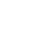# Henry's Law Constants

### Rolf Sander

Atmospheric Chemistry Division

Max-Planck Institute for Chemistry
Mainz, Germany

## Errata

#### Contact, Impressum, Acknowledgements

When referring to the compilation of Henry's Law Constants, please cite this publication:

R. Sander: Compilation of Henry's law constants (version 4.0) for water as solvent, Atmos. Chem. Phys., 15, 4399-4981 (2015), doi:10.5194/acp-15-4399-2015

# Henry's Law Constants → Inorganic species → Rare gases (He, Ne, Ar, Kr, Xe, Rn) → helium

 FORMULA: He CAS RN: 7440-59-7 STRUCTURE (FROM NIST):InChIKey: SWQJXJOGLNCZEY-UHFFFAOYSA-N

$Hscp$ $d ln Hs cp / d (1/T)$ Reference Type Notes
[mol/(m3Pa)] [K]
3.9×10−6 15 Fernández-Prini et al. (2003) L 1)
3.8×10−6 83 Abraham and Matteoli (1988) L
3.8×10−6 92 Wilhelm et al. (1977) L
3.9×10−6 69 Krause and Benson (1989) M
3.7×10−6 360 Morrison and Johnstone (1954) M
3.8×10−6 83 Clever (1979a) X 3) 80)
3.8×10−6 120 Clever (1979a) X 5) 81)
3.7×10−6 440 Dean (1992) ? 6)
3.8×10−6 Abraham et al. (1990) ?

## References

• Abraham, M. H. & Matteoli, E.: The temperature variation of the hydrophobic effect, J. Chem. Soc. Faraday Trans. 1, 84, 1985–2000, doi:10.1039/F19888401985 (1988).
• Abraham, M. H., Whiting, G. S., Fuchs, R., & Chambers, E. J.: Thermodynamics of solute transfer from water to hexadecane, J. Chem. Soc. Perkin Trans. 2, pp. 291–300, doi:10.1039/P29900000291 (1990).
• Clever, H. L., ed.: IUPAC Solubility Data Series, vol. 1 of Helium and Neon, Pergamon Press, Oxford, England (1979a).
• Dean, J. A.: Lange’s Handbook of Chemistry, McGraw-Hill, Inc. (1992).
• Fernández-Prini, R., Alvarez, J. L., & Harvey, A. H.: Henry’s constants and vapor-liquid distribution constants for gaseous solutes in H2O and D2O at high temperatures, J. Phys. Chem. Ref. Data, 32, 903–916, doi:10.1063/1.1564818 (2003).
• Krause, Jr., D. & Benson, B. B.: The solubility and isotopic fractionation of gases in dilute aqueous solution. IIa. solubilities of the noble gases, J. Solution Chem., 18, 823–873, doi:10.1007/BF00685062 (1989).
• Morrison, T. J. & Johnstone, N. B.: Solubilities of the inert gases in water, J. Chem. Soc., pp. 3441–3446, doi:10.1039/JR9540003441 (1954).
• Wilhelm, E., Battino, R., & Wilcock, R. J.: Low-pressure solubility of gases in liquid water, Chem. Rev., 77, 219–262, doi:10.1021/CR60306A003 (1977).

## Type

Table entries are sorted according to reliability of the data, listing the most reliable type first: L) literature review, M) measured, V) VP/AS = vapor pressure/aqueous solubility, R) recalculation, T) thermodynamical calculation, X) original paper not available, C) citation, Q) QSPR, E) estimate, ?) unknown, W) wrong. See Section 3.1 of Sander (2015) for further details.

## Notes

 1) Vapor pressure data for water from Wagner and Pruss (1993) was needed to calculate Hs. 3) Value given here as quoted by Fogg and Sangster (2003). 5) Value given here as quoted by Lide and Frederikse (1995). 6) Only the tabulated data between T = 273 K and T = 303 K from Dean (1992) were used to derive Hs and its temperature dependence. Above T = 303 K, the tabulated data could not be parameterized very well. The partial pressure of water vapor (needed to convert some Henry's law constants) was calculated using the formula given by Sander et al. (1995). The quantities A and α from Dean (1992) were assumed to be identical. 80) Erratum for page 265 of Fogg and Sangster (2003): The corresponding equation is incorrect. The second term should not be divided by 100 K. 81) The value at T = 308.15 K doesn't fit and is not used for the linear regression.

The numbers of the notes are the same as in Sander (2015). References cited in the notes can be found here.

# * * *

## Convert Henry's Law Constants

Convert: mol/(m3*Pa) (Hscp) M/atm (Hscp) 1/atm (Hsxp) mol/(kg*Pa) (Hsbp) mol/(kg*atm) (Hsbp) (dimensionless aq/g) (Hscc) m3*Pa/mol (Hvpc) m3*atm/mol (Hvpc) atm (Hvpx) (dimensionless g/aq) (Hvcc) (dimensionless aq/g) (alpha)